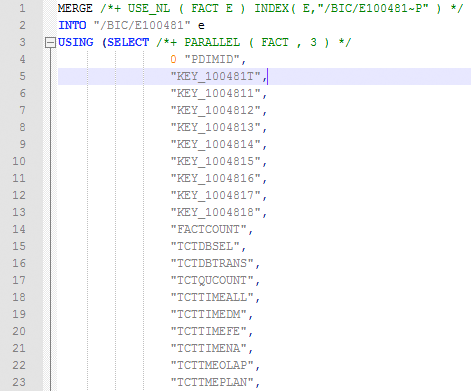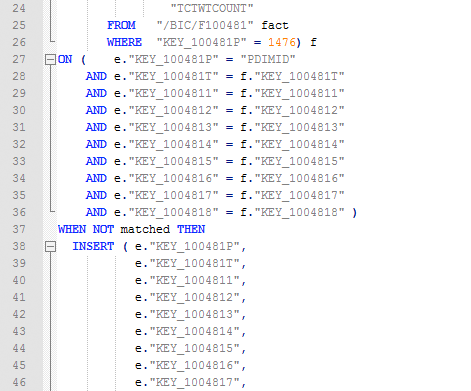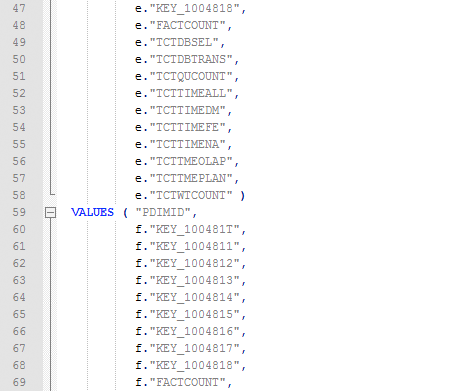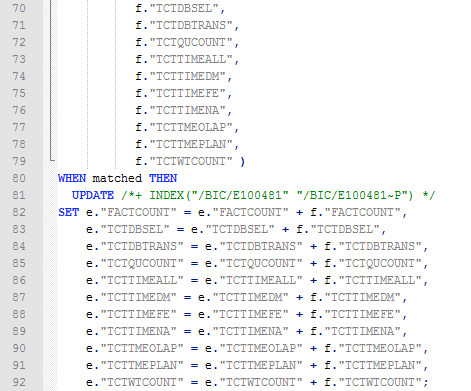# BW/DB Support 101 – Get to know your failing SQL statement

Lately, I’ve been confronted with several customer messages concerning problems with Infocube compression, change runs or roll-ups.
For some reason, these standard BW actions, that worked the day before, now started to fail.

The first step in cases like these is, of course, to find out, why the actions fail.
The usual starting point for the investigation besides the application messages or logs is the system log (SM21).

In most cases I found messages like the following in the system log:

```
Time     Type Nr  Clt User       TCode   Priority Grp N Text
[...]
<strong>21:52:46</strong> BTC  <strong>020</strong> 001 ALEREMOTE                   BY  2 Database error 12801 at EXE
21:52:46 BTC  020 001 ALEREMOTE                   BY  0 &gt; <strong>ORA-12801</strong>: error signaled in parallel query server
21:52:46 BTC  020 001 ALEREMOTE                   BY  0 &gt; P000#<strong>ORA-01438</strong>: value larger than specified precision allowe
21:52:46 BTC  020 001 ALEREMOTE                   BY  0 &gt; for this column
21:52:46 BTC  020 001 ALEREMOTE                   BY  1 Database error 12801
[...]

There was a database problem for the batch work process nr. 20 short before 10 at night. And we can already see what kind of problem there was, but we've to have a sharp eye to see that there are in fact two error messages present here:```
```ORA-12801: error signaled in parallel query server P000
ORA-01438: value larger than specified precision allowed for this column```

The first line tells us, that there was a problem during a parallel query that led to the abortion of one of the parallel query server processes, P000 in this case.
The second line gives us more information about the kind of problem: it was tried to insert or update a column in a table with a value that exceeds the data type definition of this column.
So, this is the root cause of the compression run to fail.
There was some kind of overflow.
But in which table and for which column?

For that to know, we must dig deeper and take a look at the work process developer trace for work process 20.
In transaction ST11 we open the file dev_w20 and look out for our error by searching for the timestamp “21:52:46”.
Sure enough, we find this in there:

```
C Wed Feb  2 21:52:46 2011
C     OCIStmtExecute() failed with -1=OCI_ERROR
C     SQL error 12801:
C  *** ERROR =&gt; Error 12801 in stmt_execute() from oci_execute_stmt(), orpc=0
[dbsloci.c    13103]
C  *** ERROR =&gt; ORA-12801 occurred when executing SQL stmt (parse error offset=0)
[dbsloci.c    13105]
C  sc_p=0x10751b950,no=214,idc_p=(nil),con=0,act=1,slen=2245,smax=2304,#vars=0,stmt=0x1094c2cb0,table=
C  MERGE/*+ USE_NL ( FACT E ) INDEX( E,"/BIC/E100481~P" ) */ INTO "/BIC/E100481" E USING ( SELECT  /*+
C  PARALLEL ( FACT , 3 ) */0 "PDIMID" , "KEY_100481T" , "KEY_1004811" , "KEY_1004812" , "KEY_1004813" ,
C   "KEY_1004814" , "KEY_1004815" , "KEY_1004816" , "KEY_1004817" , "KEY_1004818" , "FACTCOUNT" , "TCTD
C  BSEL" , "TCTDBTRANS" , "TCTQUCOUNT" , "TCTTIMEALL" , "TCTTIMEDM" , "TCTTIMEFE" , "TCTTIMENA" , "TCTT
C  MEOLAP" , "TCTTMEPLAN" , "TCTWTCOUNT" FROM "/BIC/F100481" FACT WHERE "KEY_100481P" =1476 ) F ON ( E.
C  "KEY_100481P" = "PDIMID" AND E."KEY_100481T" = F."KEY_100481T" AND   E."KEY_1004811" = F."KEY_100481
C  1" AND   E."KEY_1004812" = F."KEY_1004812" AND   E."KEY_1004813" = F."KEY_1004813" AND   E."KEY_1004
C  814" = F."KEY_1004814" AND   E."KEY_1004815" = F."KEY_1004815" AND   E."KEY_1004816" = F."KEY_100481
C  6" AND   E."KEY_1004817" = F."KEY_1004817" AND   E."KEY_1004818" = F."KEY_1004818" ) WHEN NOT MATCHE
C  D THEN INSERT ( E."KEY_100481P" , E."KEY_100481T" , E."KEY_1004811" , E."KEY_1004812" , E."KEY_10048
C  13" , E."KEY_1004814" , E."KEY_1004815" , E."KEY_1004816" , E."KEY_1004817" , E."KEY_1004818" , E."F
C  ACTCOUNT" , E."TCTDBSEL" , E."TCTDBTRANS" , E."TCTQUCOUNT" , E."TCTTIMEALL" , E."TCTTIMEDM" , E."TCT
C  TIMEFE" , E."TCTTIMENA" , E."TCTTMEOLAP" , E."TCTTMEPLAN" , E."TCTWTCOUNT" ) VALUES ( "PDIMID" , F."
C  KEY_100481T" , F."KEY_1004811" , F."KEY_1004812" , F."KEY_1004813" , F."KEY_1004814" , F."KEY_100481
C  5" , F."KEY_1004816" , F."KEY_1004817" , F."KEY_1004818" , F."FACTCOUNT" , F."TCTDBSEL" , F."TCTDBTR
C  ANS" , F."TCTQUCOUNT" , F."TCTTIMEALL" , F."TCTTIMEDM" , F."TCTTIMEFE" , F."TCTTIMENA" , F."TCTTMEOL
C  AP" , F."TCTTMEPLAN" , F."TCTWTCOUNT" ) WHEN MATCHED THEN UPDATE /*+ INDEX("/BIC/E100481" "/BIC/E100
C  481~P") */ SET E."FACTCOUNT" = E."FACTCOUNT" + F."FACTCOUNT", E."TCTDBSEL" = E."TCTDBSEL" + F."TCTDB
C  SEL", E."TCTDBTRANS" = E."TCTDBTRANS" + F."TCTDBTRANS", E."TCTQUCOUNT" = E."TCTQUCOUNT" + F."TCTQUCO
C  UNT", E."TCTTIMEALL" = E."TCTTIMEALL" + F."TCTTIMEALL", E."TCTTIMEDM" = E."TCTTIMEDM" + F."TCTTIMEDM
C  ", E."TCTTIMEFE" = E."TCTTIMEFE" + F."TCTTIMEFE", E."TCTTIMENA" = E."TCTTIMENA" + F."TCTTIMENA", E."
C  TCTTMEOLAP" = E."TCTTMEOLAP" + F."TCTTMEOLAP", E."TCTTMEPLAN" = E."TCTTMEPLAN" + F."TCTTMEPLAN", E."
C  TCTWTCOUNT" = E."TCTWTCOUNT" + F."TCTWTCOUNT";
C  sc_p=0x10751b950,no=214,idc_p=(nil),con=0,act=1,slen=2245,smax=2304,#vars=0,stmt=0x1094c2cb0,table=
C  prep=0,lit=0,nsql=1,lobret=0,#exec=1,dbcnt=0,upsh_p=(nil),ocistmth_p=0x1082889b0
C  IN : cols=0,rmax=1,xcnt=1,rpc=0,rowi=0,rtot=1,upto=4294967295,rsize=0,vmax=32,bound=0,iobuf_p=(nil),vda_p=0x108289000
C       lobs=0,lmax=0,lpcnt=0,larr=(nil),lcurr_p=(nil),rret=0
C  OUT: cols=4,rmax=16384,xcnt=0,rpc=0,rowi=0,rtot=1,upto=0,rsize=16,vmax=32,bound=0,iobuf_p=(nil),vda_p=0x108289790
C       lobs=0,lmax=0,lpcnt=0,larr=(nil),lcurr_p=(nil),rret=0
C  MERGE/*+ USE_NL ( FACT E ) INDEX( E,"/BIC/E100481~P" ) */ INTO "/BIC/E100481" E USING ( SELECT  /*+
C  PARALLEL ( FACT , 3 ) */0 "PDIMID" , "KEY_100481T" , "KEY_1004811" , "KEY_1004812" , "KEY_1004813" ,
C   "KEY_1004814" , "KEY_1004815" , "KEY_1004816" , "KEY_1004817" , "KEY_1004818" , "FACTCOUNT" , "TCTD
C  BSEL" , "TCTDBTRANS" , "TCTQUCOUNT" , "TCTTIMEALL" , "TCTTIMEDM" , "TCTTIMEFE" , "TCTTIMENA" , "TCTT
C  MEOLAP" , "TCTTMEPLAN" , "TCTWTCOUNT" FROM "/BIC/F100481" FACT WHERE "KEY_100481P" =1476 ) F ON ( E.
C  "KEY_100481P" = "PDIMID" AND E."KEY_100481T" = F."KEY_100481T" AND   E."KEY_1004811" = F."KEY_100481
C  1" AND   E."KEY_1004812" = F."KEY_1004812" AND   E."KEY_1004813" = F."KEY_1004813" AND   E."KEY_1004
C  814" = F."KEY_1004814" AND   E."KEY_1004815" = F."KEY_1004815" AND   E."KEY_1004816" = F."KEY_100481
C  6" AND   E."KEY_1004817" = F."KEY_1004817" AND   E."KEY_1004818" = F."KEY_1004818" ) WHEN NOT MATCHE
C  D THEN INSERT ( E."KEY_100481P" , E."KEY_100481T" , E."KEY_1004811" , E."KEY_1004812" , E."KEY_10048
C  13" , E."KEY_1004814" , E."KEY_1004815" , E."KEY_1004816" , E."KEY_1004817" , E."KEY_1004818" , E."F
C  ACTCOUNT" , E."TCTDBSEL" , E."TCTDBTRANS" , E."TCTQUCOUNT" , E."TCTTIMEALL" , E."TCTTIMEDM" , E."TCT
C  TIMEFE" , E."TCTTIMENA" , E."TCTTMEOLAP" , E."TCTTMEPLAN" , E."TCTWTCOUNT" ) VALUES ( "PDIMID" , F."
C  KEY_100481T" , F."KEY_1004811" , F."KEY_1004812" , F."KEY_1004813" , F."KEY_1004814" , F."KEY_100481
C  5" , F."KEY_1004816" , F."KEY_1004817" , F."KEY_1004818" , F."FACTCOUNT" , F."TCTDBSEL" , F."TCTDBTR
C  ANS" , F."TCTQUCOUNT" , F."TCTTIMEALL" , F."TCTTIMEDM" , F."TCTTIMEFE" , F."TCTTIMENA" , F."TCTTMEOL
C  AP" , F."TCTTMEPLAN" , F."TCTWTCOUNT" ) WHEN MATCHED THEN UPDATE /*+ INDEX("/BIC/E100481" "/BIC/E100
C  481~P") */ SET E."FACTCOUNT" = E."FACTCOUNT" + F."FACTCOUNT", E."TCTDBSEL" = E."TCTDBSEL" + F."TCTDB
C  SEL", E."TCTDBTRANS" = E."TCTDBTRANS" + F."TCTDBTRANS", E."TCTQUCOUNT" = E."TCTQUCOUNT" + F."TCTQUCO
C  UNT", E."TCTTIMEALL" = E."TCTTIMEALL" + F."TCTTIMEALL", E."TCTTIMEDM" = E."TCTTIMEDM" + F."TCTTIMEDM
C  ", E."TCTTIMEFE" = E."TCTTIMEFE" + F."TCTTIMEFE", E."TCTTIMENA" = E."TCTTIMENA" + F."TCTTIMENA", E."
C  TCTTMEOLAP" = E."TCTTMEOLAP" + F."TCTTMEOLAP", E."TCTTMEPLAN" = E."TCTTMEPLAN" + F."TCTTMEPLAN", E."
C  TCTWTCOUNT" = E."TCTWTCOUNT" + F."TCTWTCOUNT";
B  ***LOG BY2=&gt; sql error 12801      performing EXE        [dbds#2 @ 810] [dbds    0810 ]
B  ***LOG BY0=&gt; ORA-12801: error signaled in parallel query server P000
ORA-01438: value larger than specified precision allowed for this column [dbds#2 @ 810] [dbds    0810 ]
B  RECONNECT: added code: 3123
B  RECONNECT: added code: 3127
B  ***LOG BY1=&gt; sql error 12801      [dbacds#2 @ 1433] [dbacds  1433 ]

```

The problem with an output like this is: it’s completely unreadable for any human being.
Thus, we need to do some re-formatting to get the pure single SQL statement.
In this case, the statement starts with line

```
C  MERGE/*+ USE_NL ( FACT E ) INDEX( E,"/BIC/E100481~P" ) */ INTO "/BIC/E100481" E USING ( SELECT  /*+

```

and ends with

```
C  TCTWTCOUNT" = E."TCTWTCOUNT" + F."TCTWTCOUNT";

```

Everything else does not belong to the statement and should be removed.
The next step is to remove the three characters at the start of each line.
For that, I use the block-selection capability that some text editor programs offer.
I use the free Notepad++ for that.

With it, you can just hold down the ALT key while selecting text to use the block-selection.
Just select the first three characters of all rows and delete them (backspace key).

By now the statement should look like this:

```
MERGE/*+ USE_NL ( FACT E ) INDEX( E,"/BIC/E100481~P" ) */ INTO "/BIC/E100481" E USING ( SELECT  /*+
PARALLEL ( FACT , 3 ) */0 "PDIMID" , "KEY_100481T" , "KEY_1004811" , "KEY_1004812" , "KEY_1004813" ,
"KEY_1004814" , "KEY_1004815" , "KEY_1004816" , "KEY_1004817" , "KEY_1004818" , "FACTCOUNT" , "TCTD
BSEL" , "TCTDBTRANS" , "TCTQUCOUNT" , "TCTTIMEALL" , "TCTTIMEDM" , "TCTTIMEFE" , "TCTTIMENA" , "TCTT
MEOLAP" , "TCTTMEPLAN" , "TCTWTCOUNT" FROM "/BIC/F100481" FACT WHERE "KEY_100481P" =1476 ) F ON ( E.
"KEY_100481P" = "PDIMID" AND E."KEY_100481T" = F."KEY_100481T" AND   E."KEY_1004811" = F."KEY_100481
1" AND   E."KEY_1004812" = F."KEY_1004812" AND   E."KEY_1004813" = F."KEY_1004813" AND   E."KEY_1004
814" = F."KEY_1004814" AND   E."KEY_1004815" = F."KEY_1004815" AND   E."KEY_1004816" = F."KEY_100481
6" AND   E."KEY_1004817" = F."KEY_1004817" AND   E."KEY_1004818" = F."KEY_1004818" ) WHEN NOT MATCHE
D THEN INSERT ( E."KEY_100481P" , E."KEY_100481T" , E."KEY_1004811" , E."KEY_1004812" , E."KEY_10048
13" , E."KEY_1004814" , E."KEY_1004815" , E."KEY_1004816" , E."KEY_1004817" , E."KEY_1004818" , E."F
ACTCOUNT" , E."TCTDBSEL" , E."TCTDBTRANS" , E."TCTQUCOUNT" , E."TCTTIMEALL" , E."TCTTIMEDM" , E."TCT
TIMEFE" , E."TCTTIMENA" , E."TCTTMEOLAP" , E."TCTTMEPLAN" , E."TCTWTCOUNT" ) VALUES ( "PDIMID" , F."
KEY_100481T" , F."KEY_1004811" , F."KEY_1004812" , F."KEY_1004813" , F."KEY_1004814" , F."KEY_100481
5" , F."KEY_1004816" , F."KEY_1004817" , F."KEY_1004818" , F."FACTCOUNT" , F."TCTDBSEL" , F."TCTDBTR
ANS" , F."TCTQUCOUNT" , F."TCTTIMEALL" , F."TCTTIMEDM" , F."TCTTIMEFE" , F."TCTTIMENA" , F."TCTTMEOL
AP" , F."TCTTMEPLAN" , F."TCTWTCOUNT" ) WHEN MATCHED THEN UPDATE /*+ INDEX("/BIC/E100481" "/BIC/E100
481~P") */ SET E."FACTCOUNT" = E."FACTCOUNT" + F."FACTCOUNT", E."TCTDBSEL" = E."TCTDBSEL" + F."TCTDB
SEL", E."TCTDBTRANS" = E."TCTDBTRANS" + F."TCTDBTRANS", E."TCTQUCOUNT" = E."TCTQUCOUNT" + F."TCTQUCO
UNT", E."TCTTIMEALL" = E."TCTTIMEALL" + F."TCTTIMEALL", E."TCTTIMEDM" = E."TCTTIMEDM" + F."TCTTIMEDM
", E."TCTTIMEFE" = E."TCTTIMEFE" + F."TCTTIMEFE", E."TCTTIMENA" = E."TCTTIMENA" + F."TCTTIMENA", E."
TCTTMEOLAP" = E."TCTTMEOLAP" + F."TCTTMEOLAP", E."TCTTMEPLAN" = E."TCTTMEPLAN" + F."TCTTMEPLAN", E."
TCTWTCOUNT" = E."TCTWTCOUNT" + F."TCTWTCOUNT";

```

To do this, simple mark all lines and run two “search-and-replace” actions on this block.

1. Replace all “” with an empty string, a.k.a. nothing
2. Switch to extended search mode and replace all
with an empty string.
In Notepad++ ”
” stands for CR+LF!

Now we have the whole statement in one single line.

```MERGE/*+ USE_NL ( FACT E ) INDEX( E,"/BIC/E100481~P" ) */ INTO "/BIC/E100481" E USING ( SELECT  /*+ PARALLEL ( FACT , 3 ) */0 "PDIMID" , "KEY_100481T" , "KEY_1004811" , "KEY_1004812" , "KEY_1004813" , "KEY_1004814" , "KEY_1004815" ...
```

This is not yet better readable.
But we can use it to feed it to some automatic SQL formatting tool.
Most SQL editor programs offer this function (e.g. Oracle SQL Developer or SAP MaxDB Database Studio) and there are also free Web-tools like Instant SQL Formatter that can be used for this.

Finally, we end up with the formatted statement (due to the crappy text formatting options of the SDN blog editor I had to paste this as picture files):Now we have a large. but readable MERGE statement.
And with this statement, we can start to figure out, for which columns an overflow possible can happen.

How this is done, is explained in our new SAP note
#1557321 – Numeric Overflow during SAP BW-standard actions

Ok, I hope you liked this blog and that the re-formatting technique is useful for you the next time you’ve to analyze SQL related problems.

Best regards,
Lars